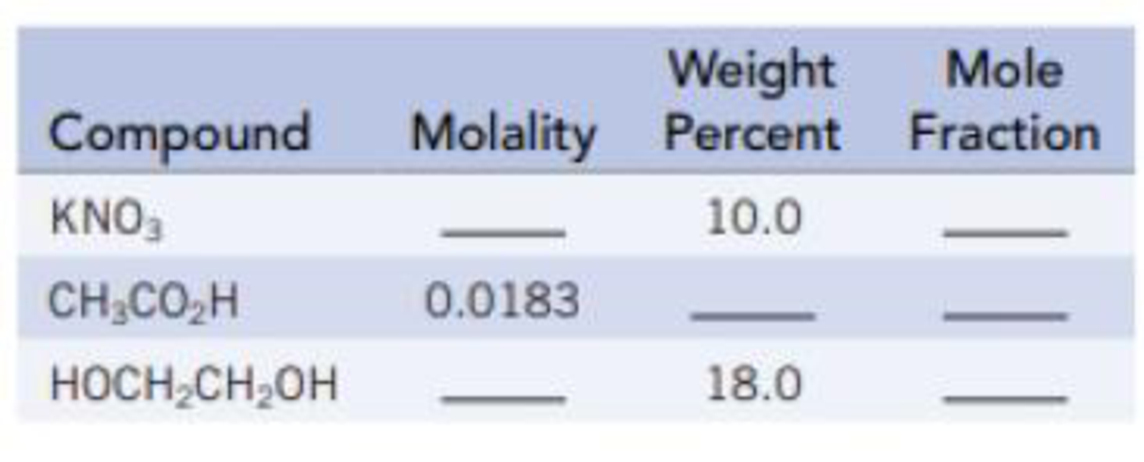Chapter 13, Problem 4PS

Chapter
Section
Textbook Problem

Fill in the blanks in the table. Aqueous solutions are assumed.Interpretation Introduction

Interpretation: The mole fraction, molality, and weight percent of given compounds to be identified.

Concept introduction:

Mole fraction: Amount of that component divided by the total amount of all of the components of the mixture ( nA +  nB +  n...).

Mole fraction of A (χA)= nA nA +  nB +  n...

Molality: The amount of solute (mol) per kilogram of solvent.

Concentration (C, mol/kg) =  molality of solute = Amount of solute (mol)Mass of solvent (Kg)

Weight percent: The mass of one component divided by the total mass of the mixture, multiplied by 100%

weight % A = Mass of A Mass of A + Mass of B + Mass of C + ...×100%

Explanation
• Calculate molality of KNO3:

Given data:

Weight percent = 10.0

Weight percent = 10.0  indictaes 10g of KNO3 in100g of solution.Mass of Water (solvent) = mass of solution - mass of KNO3 = 100 g - 10 g = 90 g

No.ofmoles=massmolarmassno.of moles (KNO3) = 10 g 101.10 g/mol = 0.099 mol

Molality of a solute is defined as the amount of solute dissolves in kilogram of solvent.

Molality=molesofsoluteMassofsolventinkg

nKNO3 ( moles of KNO3) = 0.099 molAmount of solvent = 0.090 Kg

Molality of solute:_Amount of solute (mol)Mass of solvent (Kg)0.099 mol0.090 kg = 1.14 mol/Kg = 1.1 m

The molality of KNO3 is calculated as shown above. Hence, the calculated molality of KNO31.1 m

• Calculate mole fraction of KNO3:

Known data:

Mass of water = 90 g.

No.ofmoles=massmolarmass

Moles of water=massmolarmass=90g18g/mol=5moles

nKNO3= 0.099 molnWater= 5 mol

Mole fraction of A (χA)= nA nA +  nB +  n...

(χKNO3) = nKNO3 nKNO3 +  nWater  = 0.099mol0.099 mol + 5 mol = 0.019

Convert the mass of water into unit of moles and substitute in the respective mole fraction equation as shown above. Hence, the mole fraction of KNO3 is 0.019

• Calculate Weight percentage of ethanoic acid:

Given data:

Molality=0.0183m

0.0183molofAceticacidpresentin1Kgofwater.

No.ofmoles=massmolarmass

Mass of Aceticacid = (0.0183mol)(60.05g/mol)=1.10g

Mass of water = 1000 g.

weight % A = Mass of A Mass of A + Mass of B + Mass of C + ...×100%

weight % Acetic acid = Mass of Acetic acid Mass of Acetic acid + Mass of water ×100%1.10 g(1.10g)+(1000 g)×100% = 0

Still sussing out bartleby?

Check out a sample textbook solution.

See a sample solution

The Solution to Your Study Problems

Bartleby provides explanations to thousands of textbook problems written by our experts, many with advanced degrees!

Get Started

Find more solutions based on key concepts